# 4 Descriptive Statistics

Descriptive statistics are a first step from raw data towards something more meaningful. The most common descriptive statistics either identify the middle of the data (mean, median) or how spread out the data is around the middle (percentiles, standard deviation) The statistics we calculate as descriptive statistics will be useful for many of the more advanced lessons we’ll encounter later, but they are important on their own as well.

Descriptive statistics are useful for exactly what it sounds like it would be: describing something. Specifically, describing data. Why does data need to be described? Because raw data is difficult to digest and a single data point doesn’t tell us very much.

``## Loading required package: car``
``## Loading required package: carData``
``## Loading required package: lmtest``
``## Loading required package: zoo``
``````##
## Attaching package: 'zoo'``````
``````## The following objects are masked from 'package:base':
##
##     as.Date, as.Date.numeric``````
``## Loading required package: sandwich``
``## Loading required package: survival``
``````data("CASchools")
CASchools[410,]``````
``````##     district            school       county grades students teachers
## 410    61747 Moraga Elementary Contra Costa  KK-08     1885    98.42
##     calworks  lunch computer expenditure income  english  read  math
## 410   0.0506 0.3033      241    5592.765 31.052 1.962865 697.4 695.7``````

Let’s say I have data on all schools in California. I can look at the raw data and see that Moraga Elementary has 1885 students. That’s good to know, but it raises questions. Is that a lot? A raw data point like that without context is generally useless. Someone in that school district might be able to appreciate what the number 1885 students means implicitly. You might be thinking about whether it’s larger or smaller than you remember your elementary school being, or the school of a child/relative.

Adding more raw data doesn’t necessarily help either. Let’s look at the student enrollments of all the schools.

``CASchools\$students``
``````##      195   240  1550   243  1335   137   195   888   379  2247   446
##     987   103   487   649   852   491   421  6880  2688   440   475
##    2538   476  2357  1588  7306  2601   847   452  4142  2102 10012
##    2488 25151  2267  1657   284  5370  2471 15386   184  1217  6219
##    4258  1235 16244   814 27176 10696  8935  1600  9028 10625  7151
##    2404  5804  2253  2807  3074   723  5138 20927  3017   957  1639
##    4340  5079  6639  1154   237  2987   499 11474  1088  2660   353
##     329   252   175  3835   314  4458  1313   474  1114  1358 11629
##    6195   499   417   300   457   146   460   354  1841  3760   500
##   5112   146  2141   610   337  4501  5718 19402  3401  2621   426
##    205 13668   342  6518   239  2911  6272 10218  1735   474   544
##   1987   418   196  2208  1255  1469  7114  1962  7761   216   224
##   7887   752  9328   548   104   275   443 10337   806   227  8416
##    149   220  4612   590   133  2440   133   519   222   285  3129
##   2019  5620  9775   246  7210 21338   477   727   374 18255  8787
##    797   140   235  8294  2409   150  3981  2326   501   470   575
##   3519   474   223    92  4971  2617   242   780   324   140   181
##    516   108   419 12567   287  6201   577   170   164   382  1221
##   2214  4523   793  1678   536   307   347   168   532  3272  2045
##    156  1129  3669   157  4928   103   175  4153   280   865  8735
##    412  6373   332  2903   565   586  5068   859   145   649  1789
##    775   777  3518 19294  7661   158   117   160   511  2770   551
##   5205  6437  1712   370  3182   139 11855  1068  2295  1510   579
##   1012  1212   119   590   546   248   461  6312   285  2325 11885
##    564 12380  3772   895  5714   105  1449   510   160   433  3186
##   5010   717  3548   868   507   822  1792  1202   515  1354  1252
##    823  2231   271   309  3005   966  7710   762  1708  9850   129
##  10619  4521   580  2569  6022   670 14708  6601   675  2458   144
##    573   721   992  8432   244  7116   830   160  1588  2272  1425
##    245  1349   400  4632   224   576   451   900   118  1457  4734
##   3303  6055   424  2801   187   129   188  1212  2596  4925  6257
##    868  3787  6423   678   162  8529  1862  1452   155  2536   567
##    953   296   198   218   734   189  2528  2987   208   379   145
##    706   878   594   139  2089   326   516   449   297  1579   383
##     81  5259  1960   151   946  2707   919   945   738   164   167
##    125  1091   134   600  1803   158  2392   526   141   235  3280
##   1254   948 15228    81  2768   535  2542  1940  1059  2340  3469
##   2106   478  1885  2422  1318   220   687  2341   984  3724   441
##    101  1778``````

Now we have a lot more data, but those don’t tell us much. It’s more than we can quickly interpret. We can’t judge whether Moraga is bigger than normal or smaller, because it’s hard to get a grasp of what the data is telling us or find trends with just raw figures.

We can better appreciate Moraga’s enrollment though calculating some descriptive statistics for California schools in order to supply the context. We can provide summary statistics with the command summary() and pander() to make the output more visually appealing. Pander on it’s own wont do anything, but if it is wrapped around summary we get a prettier table.

If you want to use pander in your own tables you will need to install it once on your computer install.packages(“pander”) and make sure that it’s active by running library(pander).

``````library(pander)
summary(CASchools\$students)``````
``````##    Min. 1st Qu.  Median    Mean 3rd Qu.    Max.
##    81.0   379.0   950.5  2628.8  3008.0 27176.0``````
``pander(summary(CASchools\$students))``
Min. 1st Qu. Median Mean 3rd Qu. Max.
81 379 950.5 2629 3008 27176

This is a fairly typical list of summary statistics. We should start in the middle, with two values that can be used to measure ‘central tendency’. Median and mean are both designed to help us understand the middle of the data.

## 4.1 Median

• Median. This is the middle value of your data. If you lined up 9 numbers in a row (from lowest to highest) it would be the 5th number in that line. If you had 45, it would be the 25th number. It’s always the middle value. If you have an even number, it is the average of the two middle numbers.

Let’s imagine I asked 19 students how large their family is. To calculate the median by hand, you can write the numbers in order and find the middle digit. If done with R you can use median().

``````fam <- c(1,1,1,2,2,2,2,3,3,3,3,3,4,4,4,4,5,5,5)
median(fam )``````
``##  3``

## 4.2 Mean

• Mean. This is the average value in your data. To calculate it, you add up all the individual values and divide it by the total number of observations. Or in R, you can use the command mean()
``````fam <- (1+1+1+2+2+2+2+3+3+3+3+3+4+4+4+4+5+5+5)/19
mean(fam )``````
``##  3``

## 4.3 Mode

Finally, let’s talk about a third measure of central tendancy, which doesn’t appear in the summary statistics list above. It’s used more rarely than mean or median, but it still has an important role: mode. The mode is the most frequent figure or value to appear in a list. Unfortunately, R doesn’t have a built in function that is as clear as mean() or median() to estimate the mode. I’ll create a function below to help us calculate it. Luckily, we wont be asked to calculate the mode very often.

``````fam <- (1+1+1+2+2+2+2+3+3+3+3+3+4+4+4+4+5+5+5)/19
Mode <- function(x) {
ux <- unique(x)
ux[which.max(tabulate(match(x, ux)))]
}
Mode(fam )``````
``##  3``

The mode is 3 as well. That means there were the largest number of 3’s in the data, or that 3 is the most typical number. Mean and median are more useful for numeric data, but if we had a list of words (categorical data) it would be useful. For instance, what if I surveyed my class on their favorite type of pizza?

``````pizza <- c("cheese", "pepperoni", "pepperoni", "hawaiian", "cheese", "cheese",
"pepperoni", "pepperoni", "supreme", "pepperoni","supreme")
mean(pizza)``````
``````## Warning in mean.default(pizza): argument is not numeric or logical:
## returning NA``````
``##  NA``
``Mode(pizza )``
``##  "pepperoni"``

We get an error message when we try to take the mean because there is no average answer. There is a most common answer though: pepperoni. If I pick a student at random, the most likely favorite pizza type is pepperoni, even if that isn’t the pick of the majority.

Sometimes, like in the examples above about family size, the mean, median, and mode are the same thing. Why do we need multiple measures then? Because they often aren’t the same, and that tells us something important about the data.

In the California school data the median was 950.5 and the mean was 2629. That is a sizable gap. And the school we started with, Morgana, is larger than the median but smaller than the mean. What does that indicate? It means the data is skewed.

Let’s look back at data we made up earlier for the size of families.

``````fam <- c(1,1,1,2,2,2,2,3,3,3,3,3,4,4,4,4,5,5,5)
barplot(table(fam), ylim=c(0, 8))
abline(v=3.1, col="blue", lty=3, lwd=4)
text(3.6, 6, "Median = 3")
text(2.6, 6, "Mean = 3")``````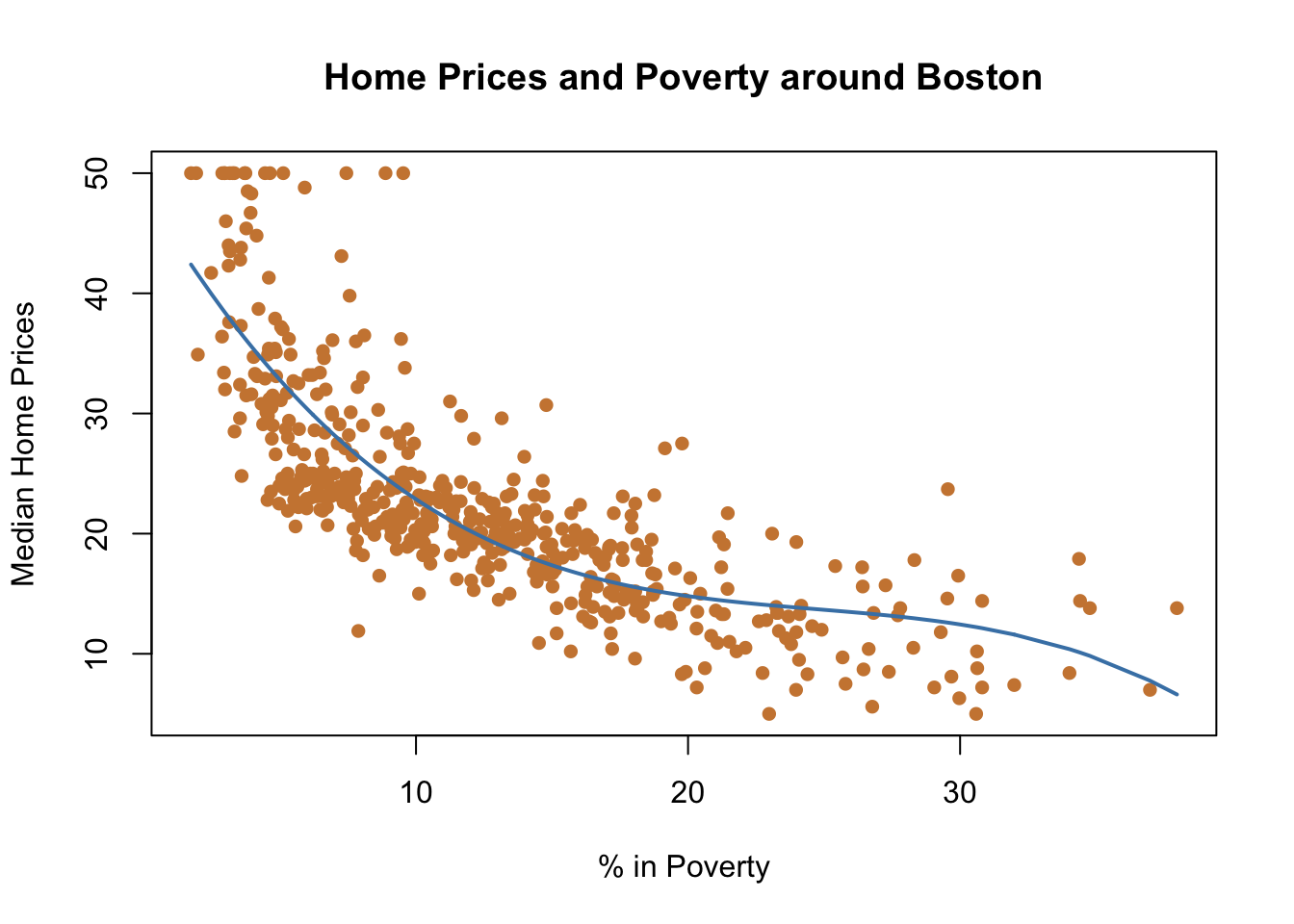Here, half of the data falls above the mean/median, and half below. If half the data is above and below the mean (or if it is close), the median and mean will tell a very similar story.

But we didn’t get similar results in California. Let’s look at the distribution for the data on student enrollment.

``````barplot(table(round(CASchools\$students, -2)), ylim=c(0, 80))
text(13, 65, "Median = 950.5")
text(13, 60, "Mean = 2627")
segments(2, 0, 2, 60, col="red", lty=3, lwd=4)
segments(3.5,  0, 3.5, 56, col="blue", lty=3, lwd=4)``````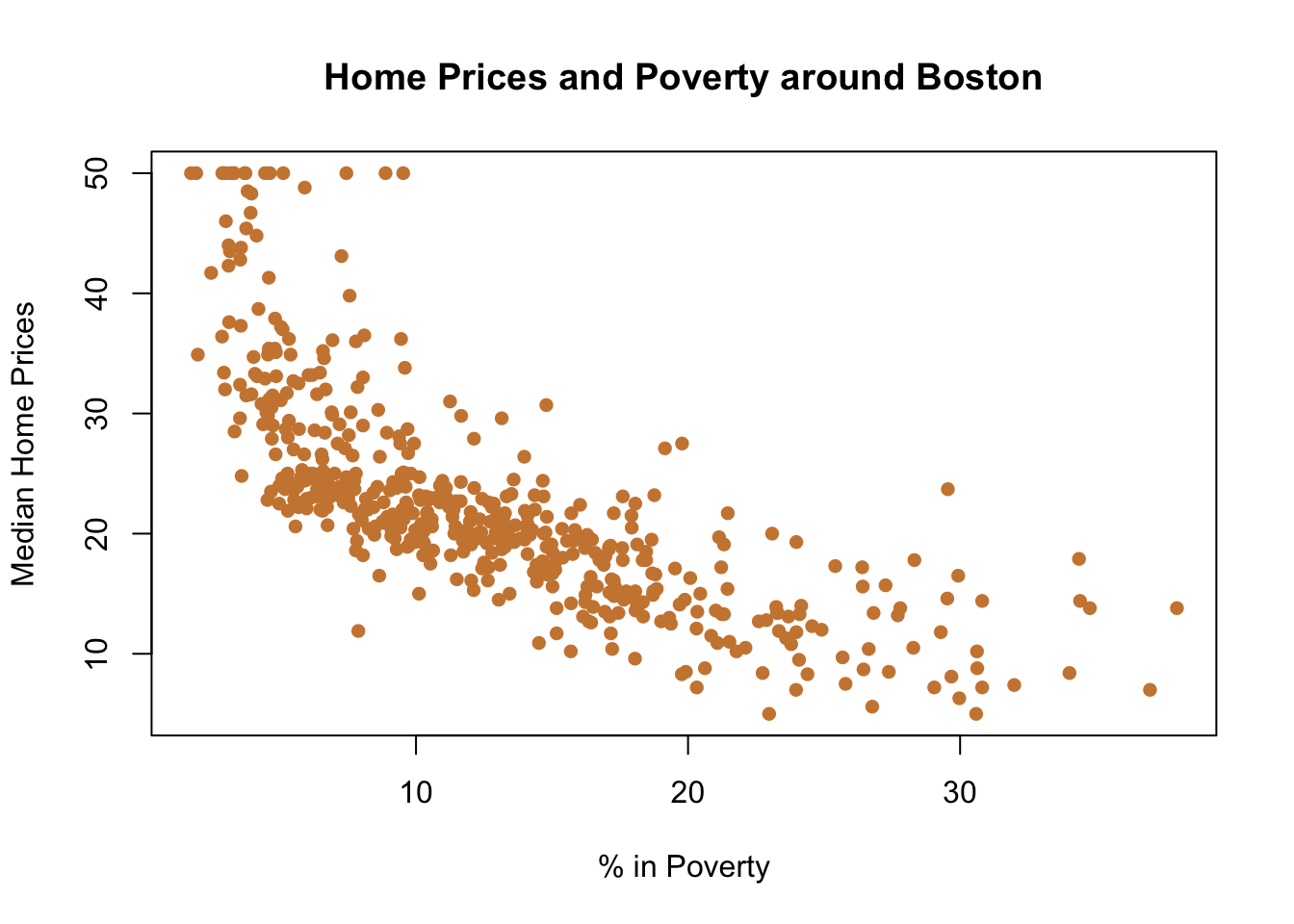## 4.4 Skew

That doesn’t look evenly distributed at all. Rather it’s skewed. Skew just means not symmetrical, which in this context means that the distribution doesn’t fall evenly around the mean and median like our earlier example. It’s heavily skewed to the right, or dragged out to that end. There’s a long tail of data that is much larger than the mean and median. Those big schools have a large impact on the mean, but less of one on the median. To illustrate that, let’s say I ask two more people about their families, and they both have a lot of relatives (17 and 19).

``````fam <- c(1,1,1,2,2,2,2,3,3,3,3,3,4,4,4,4,5,5,5,17,19)
mean(fam )``````
``##  4.428571``
``median(fam)``
``##  3``

The median stays the same even if it’s shifted which of the 3 peoples families was the middle answer. However, the mean has increased by over 1 because of those extreme answers. The same issue is present for the schools data. While most schools are small, half have fewer than one thousand students, there are many that are very large that drag the mean upwards. That isn’t bad, it’s why we have two measures of central tendency.

So which one is best? To some degree, it depends on what you’re trying to answer.

If the median and mean differ significantly it is probably best to report both, or use the median. The median will always be in the middle of your data (the exact middle) so it’s a more consistent measure of central tendency.

These issues show up in the real world. What is a better measure of how the average American is doing financially, median income or mean income? Median income in 2017 was \$31,786, while the mean was \$48,150. Someone wanting to argue that Americans are doing well would probably want to highlight the mean, while those arguing for greater social protections might cite the median. With income, we have another skewed distribution, as high earners pull the data out to the right while many Americans are clustered at the other end of the distribution. It’s somewhat dated, but see the graph below for an example of how the distribution of family incomes looked in 2011.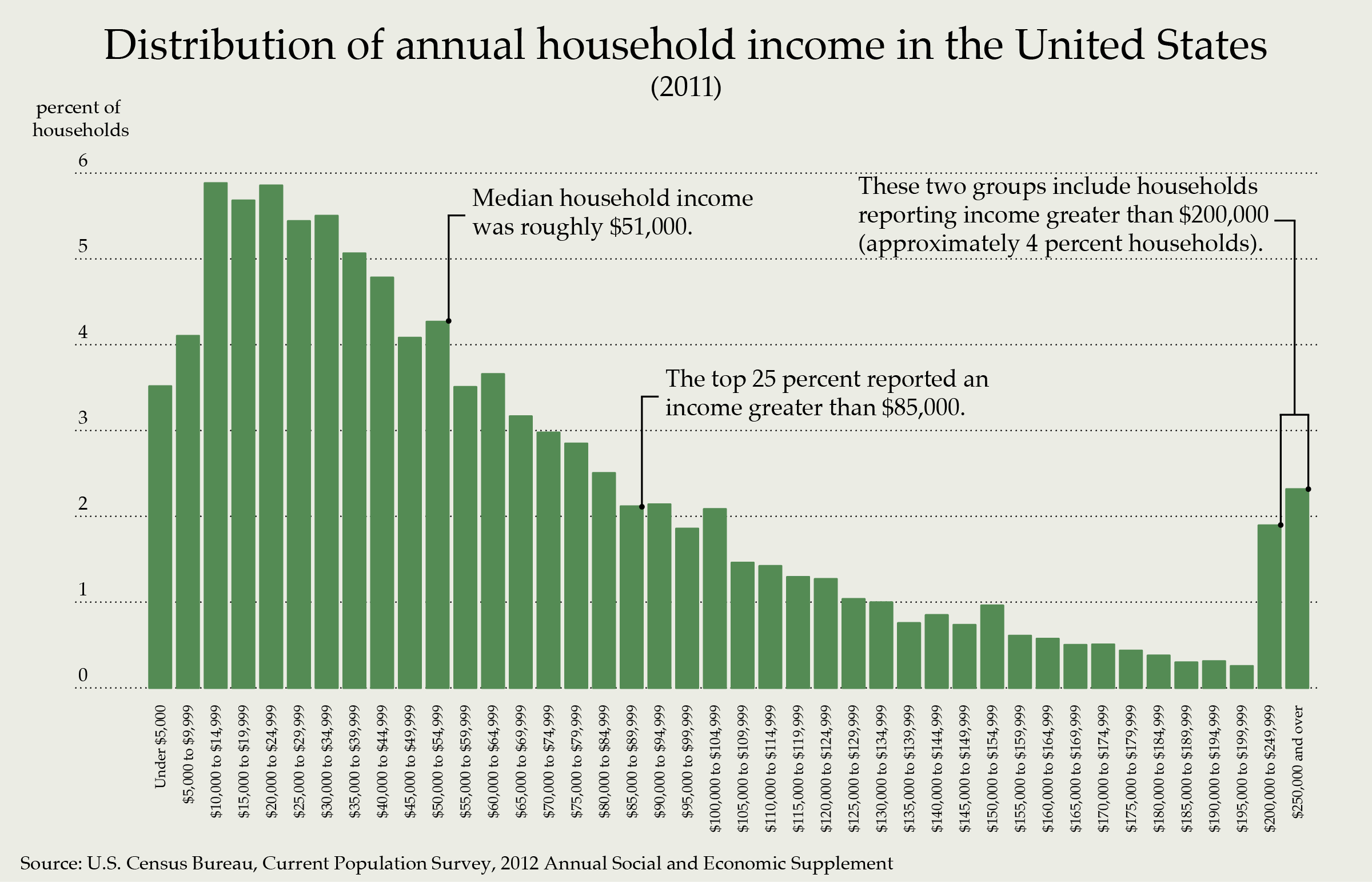wikimedia commons

## 4.5 Percentile

When we’re using skewed data, it’s really useful to report percentiles to figure out where a specific observation falls in the data. The median is the 50th percentile, because 50 percent of the data is above and below it. The 25th percentile is also known as the 1st quartile (that was in the summary statistics above) and has 25 percent of the data below it and 75 percent above it. For California schools, 25 percent of schools have 379 students or fewer, while 75 percent have more. The 3rd quartile, or 75th percentile, was 3008. That means 75 percent of schools have fewer that 3008 students, and only 25 percent have more.

Moraga had 1885 students, so it has more students than at least half the schools in California. What percent of schools does it have more than? I can add a column to the data frame (assigning it the name percentile) to figure out the percentile for each school in the data.

``````CASchools\$percentile <- ecdf(CASchools\$students)(CASchools\$students)
CASchools\$percentile``````
``##  0.6261905``

So what are percentiles good for? They take raw data points, and make them directly comparable to every other data point in a distribution. Moraga is in the 63rd percentile, so it has more students that 63 percent of California schools. It’s bigger than half of schools, but not among the largest in the state. It gives you an idea of exactly where it sits between 0 (the smallest) and 100 (the largest).

Percentiles become really useful when comparing things like test scores. You might recall percentiles from standardized tests in school, and you might take the GRE someday. The GRE is a test students take to enter graduate school and it has three parts, verbal, analytic reasoning (math), and writing. When I took the test I got a 164 out of 170 on verbal, 160 on math and a 5 out of 6 for writing. So, did I do better on the math part or the writing? Its hard to compare a score of 5 to 160. 160 is clearly larger, but is it better? Percentiles can help, because they copare my score to everyone else. I was in the 84th percentile on math and 87th on writing. Thus, I did better relative to everyone else on the writing portion.

As a quick rule, whatever percentile you have, that indicates the percentage of numbers that are below that figure. 20% are below the 20th percentile, 34% are below the 34th percentile, 79% are below the 79th percentile, and so on.

Let’s go back to our summary statistics from earlier to finish discussing all the metrics contained there.

``pander(summary(CASchools\$students))``
Min. 1st Qu. Median Mean 3rd Qu. Max.
81 379 950.5 2629 3008 27176

We’ve discussed the 1st Qu., Median, Mean, and 3rd Qu. That leaves the Min and Max, which represent the highest and lowest figures in the data. The smallest school in the data has 81 students, while the largest has 27,176 students. Those figures help us to get a feel for how spread out our data will be. The fact that the largest observation is 10 times larger than the mean indicates there are a few large observations, and a lot of small schools all clustered below the mean.

If we’re trying to understand how big the typical school is the median is probably more useful in this case. That’ll be true with most data that is skewed, or doesn’t follow a normal distribution. But in other cases the mean will be just as useful, and we’ll use it for the calculation of other statistics as well.

For instance, we’ll use the mean to calculate the standard deviation of data.

## 4.6 Standard Deviation

Standard deviation is a measure of the variability of your data. We’ve discussed two measures of the middle (mean and median), now we want to know where all the other data fall around that middle. Are they very close to the middle? Do they spread out really wide?

To calculate the standard deviation by hand we need to:

1. Calculate the mean
2. Subtract each individual observation from the mean, and square the result
3. Calculate the mean of the squared differences.
4. Calculate the square root of each figure.

That’s a mouth full. Or, we can use the command sd(). sd() takes care of all the intermediate steps outlined above.

Standard deviation indicates how dispersed your data is, or how widely it spreads around the mean. Data that has a small standard deviation generally falls very close to the mean; data with a large standard deviation is highly dispersed. The standard deviation gives you evidence of how representative the mean is of the data. If the data is very dispersed, each individual observation might be far from the mean.

Let’s imagine you’re choosing where to go for dinner. There are two new places you’ve heard about and want to check out; you look at yelp and see they have really similar ratings (out of 5). We’ll call one Oscar’s and one Luis’s (based on restaurants I like in my home town) and look at the average ratings at both.

``mean(Luis)``
``##  4.133333``
``mean(Oscars)``
``##  4.12``

That’s pretty close. It’s tough to pick between them. So you look closer and notice that Luis’s has really high variance in it’s reviews. There are a lot of 5s, but also a lot of 1s. Oscars on the other hand is more consistently rated around a 4. For Luis’s, the mean isn’t very indicative of the typical experience, but for Oscar’s you know what to expect with just that number. That’s because Luis’s data is more dispersed.

``sd(Luis)``
``##  1.547709``
``sd(Oscars)``
``##  0.6``

Why? It turns out that Luis’ brother works as a chef, and is awful. So anytime anyone rates the restaurant after eating one of the dishes cooked by him, it gets a bad review. But the other cooks are top notch. On the other hand, Oscar’s chefs are far more consistent. So the choice would depend on whether you want a chance at the better meal and are willing to take a risk on getting food poisoning, or if you’d rather just know that your food will be good - but not great.

So which restaurant do you want to go to? You plan ahead and call Luis’s to find out if his brother is working the day of your dinner, and finding out that he is home sick (he ate his own cooking apparently) you make a reservation for Luis’s.

Let’s circle back to California schools. We know how large Moraga is roughly. Let’s look at some of the other variables that are in the data.

``pander(CASchools[410,])``
Table continues below
410 61747 Moraga Elementary Contra Costa KK-08 1885
Table continues below
teachers calworks lunch computer expenditure income
410 98.42 0.0506 0.3033 241 5593 31.05
410 1.963 697.4 695.7 0.6262

Read and math refer to the average scores for the school on state achievement tests. Notice that the figure is a descriptive statistic, being the average score for students at the school. Moraga students got 695.7 on math. Is that good or bad? The numbers don’t mean anything without context. Is the score out of 696, meaning that Moraga was nearly perfect, or is it out of 10,000 and students were very not nearly perfect. Let’s look again at summary statistics for the state to try and understand.

``pander(summary(CASchools\$math))``
Min. 1st Qu. Median Mean 3rd Qu. Max.
605.4 639.4 652.4 653.3 665.8 709.5

Moraga scores above the mean and median for the state. That’s good, and they were also above the 3rd quartile, meaning that at least 75 percent of schools performed worse.

Notice that the median and mean for this figure are very close together. That means reporting either statistics will be good enough, and that the data isn’t skewed in either direction. The data forms what we would call a normal distribution (we used that term above). Let’s look at the data for math scores in a graph.

``hist(CASchools\$math, breaks=25, col="steelblue")``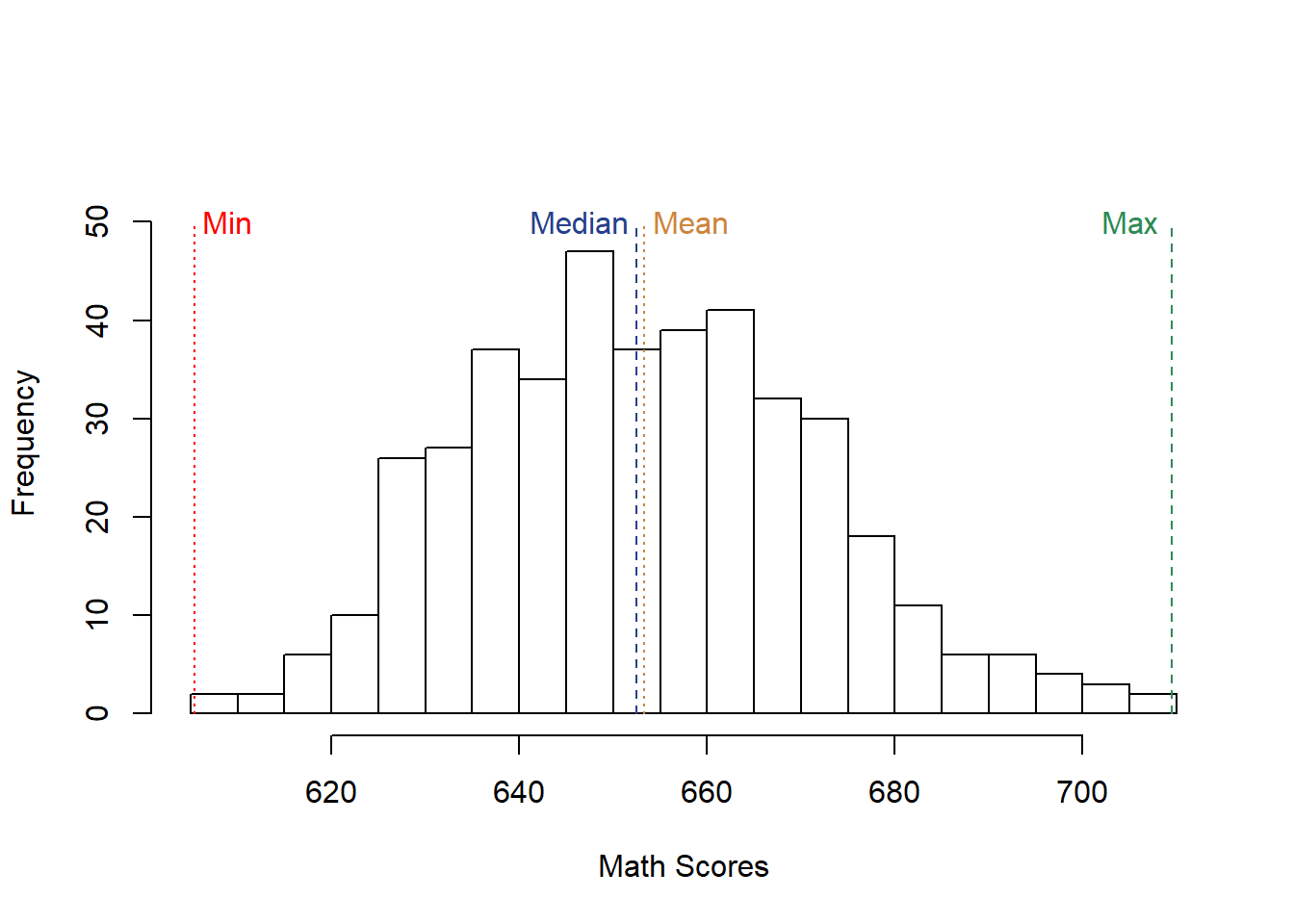You can see the outline of a bell there, even if it’s still imperfect. That’s really useful, because it means that roughly half of the observations fall above and below the mean. That means we can talk about the distance of an individual school from the average in standard units with the standard deviation.

We know Moraga did well on the tests, but how well?

``695.7-653.3``
``##  42.4``

They scores 42 points higher than the average school, but that doesn’t help us understand just how well they did. To get a better idea, we need to know how widely school math scores were distributed, to better appreciate how much better 42 points makes a school.

``sd(CASchools\$math)``
``##  18.7542``

The standard deviation is 18.7. It probably doesn’t feel like that tells you much yet, but it will. The great thing about standard deviation is that when they’re taken for normally distributed data (like average math scores in California schools) we can use them to figure out just how above or below average a given school is.

That’s because 50% of the data falls above and below the mean, and the same is true for the standard deviations. But the data also falls above and below the mean in a specific form or shape.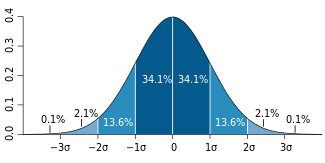credit: Wikipedia

50 percent of the data is below and above the mean in the figure above, which has a mean of 0 (the Greek character  means standard deviation). But just as importantly, we know where that 50 percent falls. 34.1 percent of the data falls within 1 standard deviation. 13.6 percent falls between 1 and 2 standard deviations, and 47.7 falls between the mean and 2 standard deviations. And those figures are symmetrical on both sides. That might not seem exciting yet, but let’s go back to our earlier question. How good at math is Moraga. First, let’s see how many standard deviations it is above the mean for math scores. We need to find the difference between the Moraga score and the average score for the state, and divide that by the standard deviation.

``(695.7-mean(CASchools\$math))/sd(CASchools\$math)``
``##  2.258554``

Moraga is 2.25 standard deviations above the mean, which shows that it did better than 95% of schools in the state. That’s pretty good.

That may all sound a lot like the percentiles that we calculated earlier. In fact, Moraga is in the 98 percentile for math scores. So why do we need two figures? Percentiles are more flexible, and can be useful for any data no matter the distribution.

However, standard deviation is useful for undersatnding how far from average a result is. Units refers to how we measure something, whether it be students, or math scores, or shoes, or dollars, or anything.

Moraga is 42 points above average in math scores, and their parents reported 15,735 more dollars than average in annual income. Which of those numbers is more impressive or further from the mean? Well, 15,735 is larger than 42, but because income and math scores are in different units, it’s a lot like comparing apples to pterodactyls. But standard deviations allow us to figure out the relative distance both have from the means.

``(695.7-mean(CASchools\$math))/sd(CASchools\$math)``
``##  2.258554``
``(31.052-mean(CASchools\$income))/sd(CASchools\$income)``
``##  2.177644``

They’re both well above average, but the math score is slightly more above average.

In addition to comparing different units, standard errors are used in calculating a lot of the tests we use to determine whether numbers are meaningful. It will come up a lot in future chapters, so understanding the basic idea as a descriptive statistic is worth your time.

In this chapter, we’ve gone over one way to summarize data and to make raw data and figures more understandable for ourselves and others. Let’s review the R commands we’ve done in this chapter.

We can call in data that is already loaded into r with data(). Let’s use a data set about Arrest rates in American states.

``data("USArrests")``

To see the top 5 lines of the data we can use the command head() or to see the bottom we can use tail()

``head(USArrests)``
``````##            Murder Assault UrbanPop Rape
## Alabama      13.2     236       58 21.2
## Alaska       10.0     263       48 44.5
## Arizona       8.1     294       80 31.0
## Arkansas      8.8     190       50 19.5
## California    9.0     276       91 40.6
## Colorado      7.9     204       78 38.7``````
``tail(USArrests)``
``````##               Murder Assault UrbanPop Rape
## Vermont          2.2      48       32 11.2
## Virginia         8.5     156       63 20.7
## Washington       4.0     145       73 26.2
## West Virginia    5.7      81       39  9.3
## Wisconsin        2.6      53       66 10.8
## Wyoming          6.8     161       60 15.6``````

We can use summary to view the descriptive statistics we went over earlier

``summary(USArrests)``
``````##      Murder          Assault         UrbanPop          Rape
##  Min.   : 0.800   Min.   : 45.0   Min.   :32.00   Min.   : 7.30
##  1st Qu.: 4.075   1st Qu.:109.0   1st Qu.:54.50   1st Qu.:15.07
##  Median : 7.250   Median :159.0   Median :66.00   Median :20.10
##  Mean   : 7.788   Mean   :170.8   Mean   :65.54   Mean   :21.23
##  3rd Qu.:11.250   3rd Qu.:249.0   3rd Qu.:77.75   3rd Qu.:26.18
##  Max.   :17.400   Max.   :337.0   Max.   :91.00   Max.   :46.00``````

In order to make a more attractive table in Markdown, we want to use pander(). We need to load pander in though, because it’s an additional package. If you haven’t loaded in before use install.packages(“pander”) before calling it into use with library(pander)

``````library(pander)
pander(summary(USArrests))``````
Murder Assault UrbanPop Rape
Min. : 0.800 Min. : 45.0 Min. :32.00 Min. : 7.30
1st Qu.: 4.075 1st Qu.:109.0 1st Qu.:54.50 1st Qu.:15.07
Median : 7.250 Median :159.0 Median :66.00 Median :20.10
Mean : 7.788 Mean :170.8 Mean :65.54 Mean :21.23
3rd Qu.:11.250 3rd Qu.:249.0 3rd Qu.:77.75 3rd Qu.:26.18
Max. :17.400 Max. :337.0 Max. :91.00 Max. :46.00

in order to just calculate the mean or median for one column we can use mean() or median(), in order to measure the middle of the data.

``mean(USArrests\$Murder)``
``##  7.788``
``median(USArrests\$Murder)``
``##  7.25``

And finally, we can calculate the dispersion or standard deviation with sd()

``sd(USArrests\$Murder)``
``##  4.35551``

## 4.7 Video Coding Review

In the following two videos, I go over a few of the basic commands done above, but focus largely just on the coding aspects of this chapter.